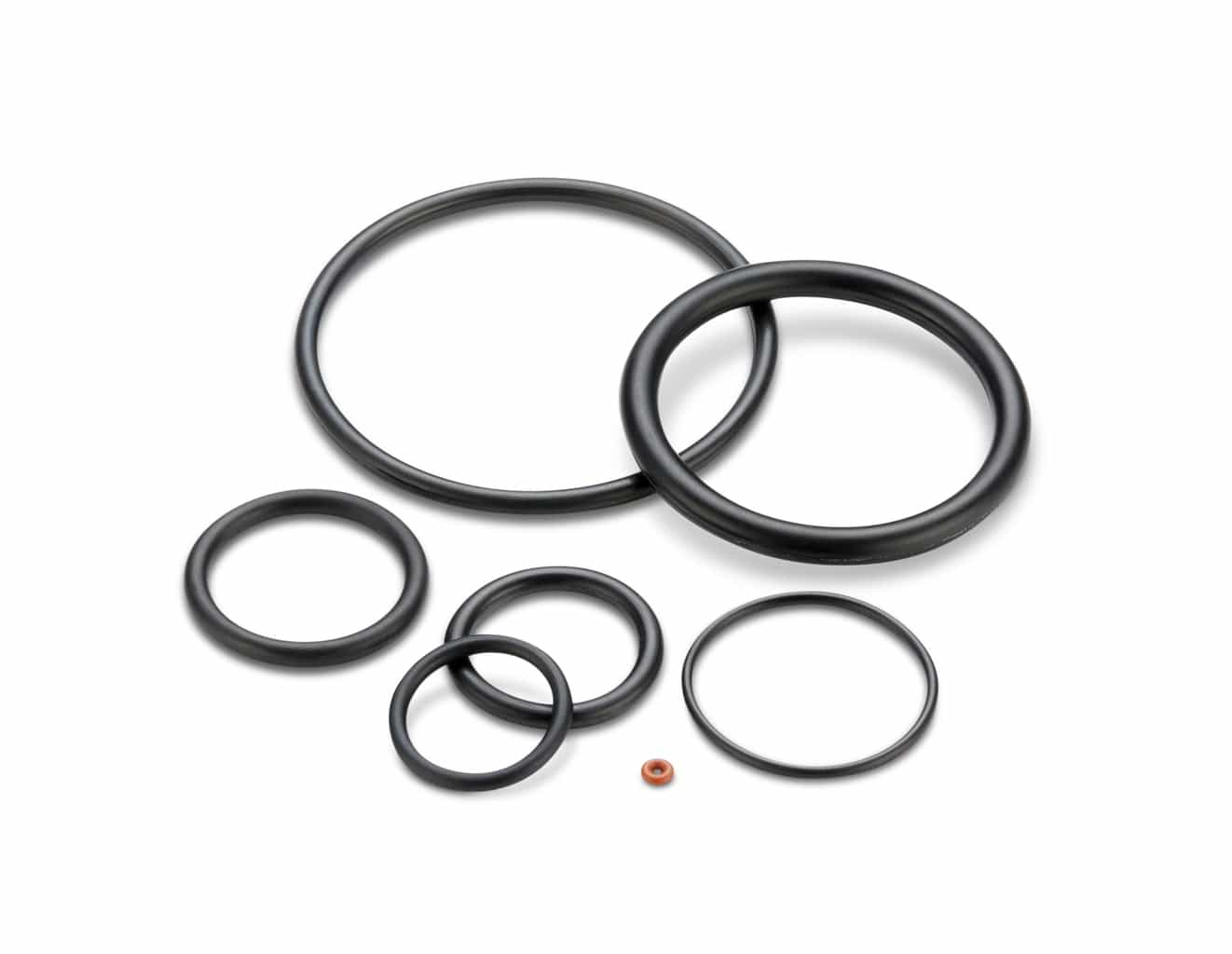# Rod Seal Application Example

## Application Example: Rod Quad-Ring® Brand Seal

#### Application description: Water faucet valve, U. S. Customary Units (inches)

• 0.25″ dynamic stroke
• Rod (shaft) diameter: 0.374″ ±0.003
• Bore diameter: 0.385″ ±0.003
• 150 psi maximum pressure
• 0.070″ cross-section Quad-Ring® Brand seal

1. Calculate the Seal Groove Diameter:
Groove Diameter
= Min Shaft Diameter + (2 X Dynamic Gland Depth)
= 0.371 + ( 2 X 0.061)
= 0.493 +0.000 / -0.002
(Recall the gland depth values in the chart are given as radial values)

2. From the chart, the groove width is 0.080 -0.000/+0.005

3. Calculate the Minimum Gland Cross-sectional Area:
Minimum Gland Volume
= ((Min Groove Dia – Max Rod Dia. / 2) X Min Groove Width
= ((0.491 – 0.377 ) / 2) X 0.080
= 0.00456 in2

4. Calculate the Maximum Quad-Ring® Brand Seal‚ Cross-sectional Area:
= (Max Quad-Ring® Brand Cross-section)2 X 0.8215
= (0.073)2 X 0.8215
= 0.0044 in2

5. Compare the Minimum Gland Volume to the Maximum Quad-Ring® Brand Volume
In this application the maximum seal volume is less than the minimum gland volume, so the seal should function satisfactorily.

6. Calculate the Minimum and Maximum Seal Squeeze
a. Max Seal Squeeze = 1 – (Min Gland Depth / Max Seal Cross-section)
Min Gland Depth = (Min Groove Dia. – Max Rod Dia.) / 2
= (0.491 – 0.377) / 2
= 0.057
Max Seal Squeeze = 1 – (0.057 /0.073)
= 0.219
= 21.9%
b. Min Seal Squeeze = 1 – (Max Gland Depth / Min Seal Cross-section)
Max Gland Depth = (Max Groove Dia. – Min Rod Dia.)
= (0.493 – 0.371) / 2
= 0.061
Min Seal Squeeze = 1 – (0.061/0.067)
= 0.09
= 9.0%
Therefore, sufficient squeeze should exist to seal this application.

7. Calculate the Maximum Clearance and evaluate possible extrusion problems
Max Radial Clearance = (Max Bore Dia. – Min Rod Dia.) / 2
= (0.388 – 0.371) / 2
= 0.0085
From the Clearance Chart, the recommended maximum radial clearance for a Quad-Ring® Brand seal with a hardness of 70 Shore A at 150 psi is slightly greater than 0.009 inches. The seal should work in this application.

8. Select the Seal Size
Refer to the Selection Guide and turn to the section which lists the seals having a 0.070 cross-section. This example’s rod size of 0.374 is very close to the standard size of 0.375, so the standard seal for a 0.375 rod will probably work. Since in this application the sealing is occurring on the rod, use the Rod column to look up the seal size for a 0.375 rod. The correct seal is a number 4 -012 (with the 4 prefix signifying a Quad-Ring® Brand seal). Note the seal inside diameter, which is 0.364 ±0.005. This will be used below.

9. Calculate the Installed Seal Stretch
Stretch % = ((Installed Seal ID – Original Seal Inside Diameter) / Original Seal Inside Diameter) x 100
= ((Rod Diameter – Original Seal Inside Diameter) / Original Seal Inside Diameter) x 100
= ((0.374 – 0.364) / 0.364) x 100
= (0.010 / 0.364) x 100 = 2.7 %